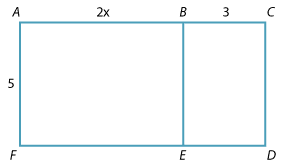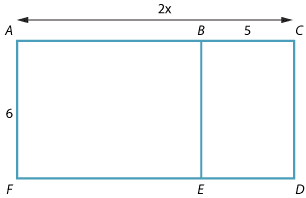The distributive law can be illustrated using diagrams.

#### Example 1Area of rectangle ACDF = area of rectangle ABEF + area of rectangle BCDE.

\begin{align*} \text{That is, }5(2x+3)&= 5\times2x+5\times3\\\\ &= 10x+15 \end{align*}

#### Example 2Area of rectangle ABEF = area of rectangle ACDF − area of rectangle BCDE.

\begin{align*} \text{That is, }6(2x-5)&= 6\times2x-6\times5\\\\ &= 12x+30 \end{align*}

### Summary

In general, $$a(b+c)=ab+ac\ \text{ and }\ a(b–c)= ab\ –\ ac$$.

Each term in the brackets is multiplied by the term outside the bracket.